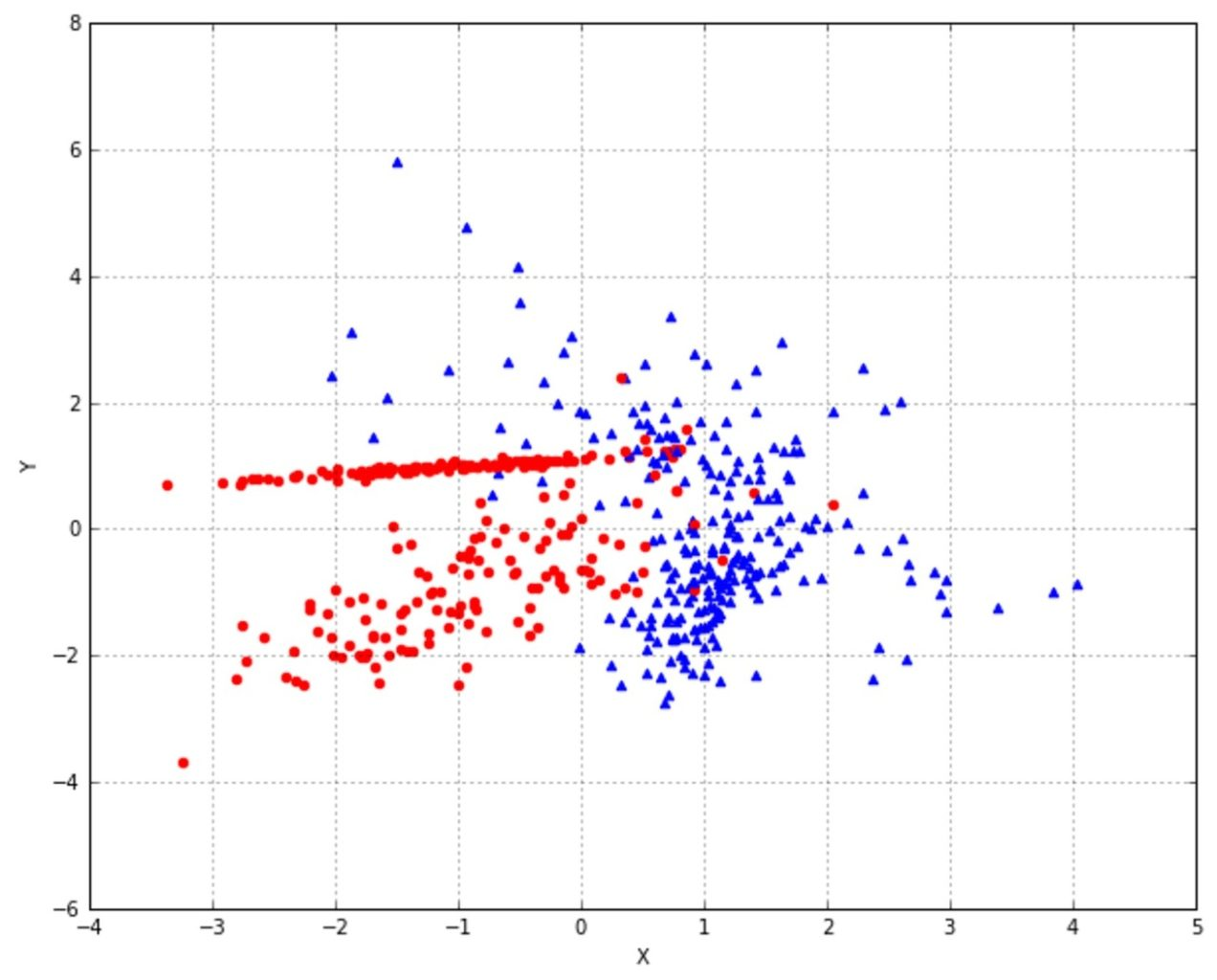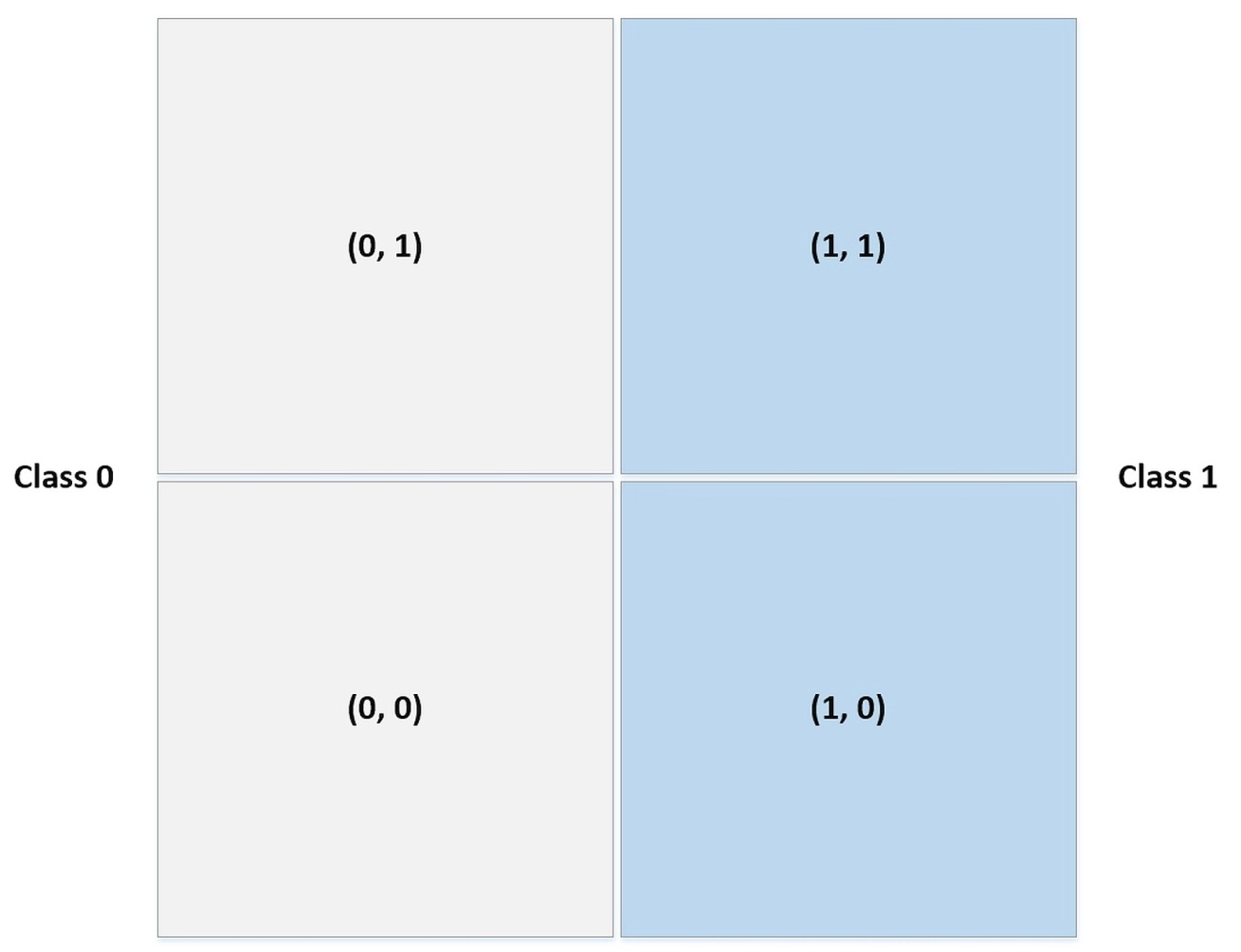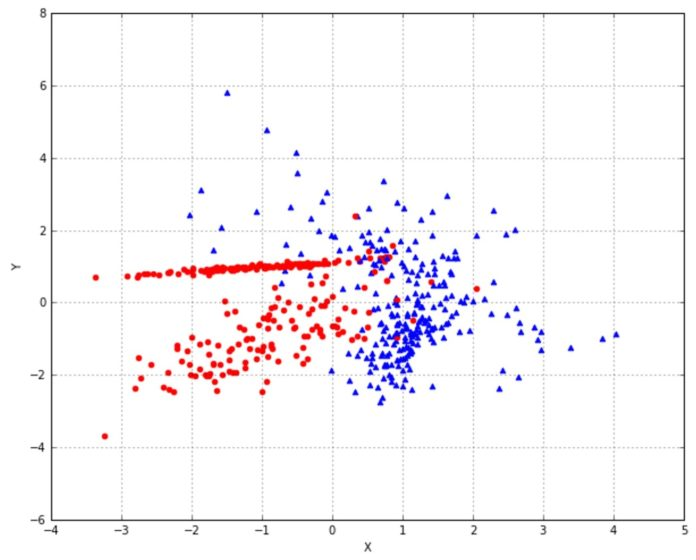• Mentors

# Naive Bayes Classification Using Scikit-learn In PythonSakshi Gupta | 6 minute read | October 27, 2021Data Classification is one of the most common problems to solve in data analytics. While the process becomes simpler using platforms like R & Python, it is essential to understand which technique to use. In this blog post, we will speak about one of the most powerful & easy-to-train classifiers, ‘Naive Bayes Classification. This is a classification technique that determines the probability of an outcome, given a set of conditions using the Bayes theorem.

We have studied its possible applications and even tried our hand at the email spam filtering dataset on Python. One of the most important libraries that we use in Python, the Scikit-learn provides three Naive Bayes implementations: Bernoulli, multinomial, and Gaussian.

Before we dig deeper into Naive Bayes classification in order to understand what each of these variations in the Naive Bayes Algorithm will do, let us understand them briefly…

• Bernoulli’s is a binary algorithm particularly useful when a feature can be present or not.
• Multinomial Naive Bayes assumes a feature vector where each element represents the number of times it appears (or, very often, its frequency).
• The Gaussian Naive Bayes, instead, is based on a continuous distribution characterised by mean & variance. It is suitable for more generic classification tasks.

Let’s dig into each of these techniques, and see the best use of them in our data analytics problems…

## Naive Bayes Classification Using Bernoulli

If ‘A’ is a random variable then under Naive Bayes classification using Bernoulli distribution, it can assume only two values (for simplicity, let’s call them 0 and 1). Their probability is:

`P(A) = p  if A = 1P(A) = q  if A = 0Where q = 1 - p & 0 < p < 1`

Let’s try this algorithm on a dummy dataset that we create. We will use the scikit-learn library to implement the Bernoulli Naive Bayes algorithm. Do remember, Bernoulli naive Bayes expects binary feature vectors, however, the class Bernoulli Naive Bayes Algorithm has a binarize parameter. This parameter allows specifying a threshold that will be used internally to transform the features:

`from sklearn.datasets import make_classification>>> nb_samples = 300>>> X, Y = make_classification(n_samples=nb_samples, n_features=2, n_informative=2, n_redundant=0)`

It generates a bidimensional dataset as below:This image is created after implementing the code Python

We have decided to use 0.0 as a binary threshold. This way, each point can be characterised by the quadrant where it’s located.

```from sklearn.naive_bayes import BernoulliNB
from sklearn.model_selection import train_test_split
>>> X_train, X_test, Y_train, Y_test = train_test_split(X, Y, test_size=0.25)
>>> bnb = BernoulliNB(binarize=0.0)
>>> bnb.fit(X_train, Y_train)
>>> bnb.score(X_test, Y_test)
0.85333333333333339```

This score is rather good! To understand how the binary classifier worked, it’s useful to see how the data have been internally binarized:This image is created after implementing the code in Python

Let’s check the naive Bayes predictions we obtain:

`>>> data = np.array([[0, 0], [0, 1], [1, 0], [1, 1]])>>> bnb.predict(data)array([0, 0, 1, 1])`

This is the output that was expected from Bernoulli’s naive Bayes!

## Data Classification Using Multinomial Naive Bayes Algorithm

A multinomial Naive Bayes algorithm is useful to model feature vectors where each value represents the number of occurrences of a term or its relative frequency. For example, if a feature vector has n elements and each of them can assume k different values with probability pk, then:

The conditional probabilities P(xi | y) are computed with a frequency count. The frequency count corresponds to applying a maximum likelihood approach. During Multinomial Bayes Formula, Laplace smoothing factor is to be kept in mind. Its default value is 1.0 and prevents the model from setting null probabilities when the frequency is zero.

Let’s understand this with an example, using the DictVectorizer. We consider only two records: the first one representing a city, while the second one countryside.

```from sklearn.feature_extraction import DictVectorizer
>>> data = [
{'house': 100, 'street': 50, 'shop': 25, 'car': 100, 'tree': 20},
{'house': 5, 'street': 5, 'shop': 0, 'car': 10, 'tree': 500, 'river': 1}
]

>>> dv = DictVectorizer(sparse=False)
>>> X = dv.fit_transform(data)
>>> Y = np.array([1, 0])

>>> X
array([[ 100.,  100.,    0.,   25.,   50.,   20.],[  10.,    5.,    1.,    0.,    5.,  500.]])
```

Note that the term ‘river’ is missing from the first set, so it’s useful to keep alpha equal to 1.0 to give it a small probability. The output classes are 1 for city and 0 for the countryside. Now we can train a Multinomial Naive Bayes instance:

```from sklearn.naive_bayes import MultinomialNB

>>> mnb = MultinomialNB()
>>> mnb.fit(X, Y)
MultinomialNB(alpha=1.0, class_prior=None, fit_prior=True)```

To test the model, we create a dummy city with a river and a dummy country place without any river.

`>>> test_data = data = [{'house': 80, 'street': 20, 'shop': 15, 'car': 70, 'tree': 10, 'river': 1},]{'house': 10, 'street': 5, 'shop': 1, 'car': 8, 'tree': 300, 'river': 0} >>> mnb.predict(dv.fit_transform(test_data))array([1, 0])`

As we can see, this prediction is correct!

## Naive Bayes Classification Using Gaussian

Gaussian Naive Bayes is useful when working with continuous values where probabilities can be modeled using a Gaussian distribution:

The conditional probabilities P(xi | y) are also Gaussian distributed and, therefore, it’s necessary to estimate the mean and variance of each of them using the maximum likelihood approach. On considering the property of a Gaussian, we get:

• k index refers to the samples in our dataset
• P(xi|y) is a Gaussian itself

From this, we get mean and variance for each Gaussian associated with P(xi | y), & the model is hence trained.

Let’s compare Gaussian Naive Bayes with logistic regression using the ROC curves as an example.

`from sklearn.datasets import make_classification>>> nb_samples = 300>>> X, Y = make_classification(n_samples=nb_samples, n_features=2, n_informative=2, n_redundant=0)`

Here is the dataset that you may obtain:This image is created after implementing the code in Python

Let’s train both models and generate the ROC curves:

```from sklearn.naive_bayes import GaussianNB
from sklearn.linear_model import LogisticRegression from sklearn.metrics import roc_curve, auc
from sklearn.model_selection import train_test_split

>>> X_train, X_test, Y_train, Y_test = train_test_split(X, Y, test_size=0.25)
>>> gnb = GaussianNB()
>>> gnb.fit(X_train, Y_train)
>>> Y_gnb_score = gnb.predict_proba(X_test)
>>> lr = LogisticRegression()
>>> lr.fit(X_train, Y_train)
>>> Y_lr_score = lr.decision_function(X_test)
>>> fpr_gnb, tpr_gnb, thresholds_gnb = roc_curve(Y_test, Y_gnb_score[:, 1])
>>> fpr_lr, tpr_lr, thresholds_lr = roc_curve(Y_test, Y_lr_score)```

The resulting ROC curves would be like this:

As you can see, the Naive Bayes performances are slightly better than logistic regression. Both the classifiers have similar accuracy and Area Under the Curve.

`from sklearn.datasets import load_digitsfrom sklearn.model_selection import cross_val_score>>> digits = load_digits()>>> gnb = GaussianNB()>>> mnb = MultinomialNB()>>> cross_val_score(gnb, digits.data, digits.target, scoring='accuracy', cv=10).mean()0.81035375835678214>>> cross_val_score(mnb, digits.data, digits.target, scoring='accuracy', cv=10).mean()0.88193962163008377`

When trying the multinomial Naive Bayes & the Gaussian variant as well, the results come very similar. You will realise that the multinomial distribution was better fitting the data, while a Gaussian was slightly more limited by its mean and variance.

We have now understood the limitations and implications of the variations in Naive Bayes Algorithm techniques. While implementing, we need to note the possible constraints of each type, so that the algorithm generates the best outcomes.

Does this classifier algorithm solve the data problem that you have been having? If not, then check out some more techniques like k-means or knn that can help you classify data. You can learn the applications of these algorithms in Springboard’s Data Analytics Career Track Online Program. With 1:1 mentoring and project-based curriculum that comes with a job guarantee, you can kickstart your career in Data Analytics with this specially designed program. And guide you to become a successful Data Analyst.

Since you’re here…
Switching to a career in data analytics is possible, no matter your background. We’ve helped over 10,000 students make it happen. Check out our free data analytics curriculum to gauge your interest, or go all-in with our Data Analytics Bootcamp.

#### About Sakshi Gupta

Sakshi is a Senior Associate Editor at Springboard. She is a technology enthusiast who loves to read and write about emerging tech. She is a content marketer and has experience working in the Indian and US markets.